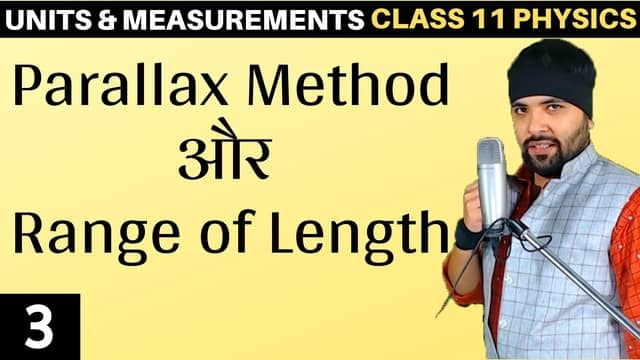# L3 – Parallax Method Units and Measurements## Topics/Questions discussed in this video:

Example 2.2 A man wishes to estimate the distance of a nearby tower from him. He stands at a point A in front of the tower C and spots a very distant object O in line with AC. He then walks perpendicular to AC up to B, a distance of 100 m, and looks at O and C again. Since O is very distant, the direction BO  is practically the same as AO; but he finds the line of sight of C shifted from the original line of sight by an angle θ = 400 (θ is known as ‘parallax’) estimate the distance of the tower C from his original position A.

Example 2.3 The moon is observed from two diametrically opposite points A and B on Earth. The angle θ subtended at the moon by the two directions of observation is 1o54′ . Given the diameter of the Earth to be about 1.276 ×107 m, compute the
distance of the moon from the Earth.

Example 2.4 The Sun’s angular diameter is measured to be 1920′′. The distance D of the Sun from the Earth is 1.496 × 1011 m. What is the diameter of the Sun ?